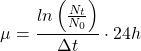# Calculate the growth rate and population doubling time of your cells

The growth rate and population doubling time is characteristic for your cells. In the exponential growth phase both parameter reach their maximum.

In this phase your cells are healthy, and they will have the best quality.

A reduction in the growth rate or increase in the population doubling time shows that the culture conditions for your cells are not ideal anymore. It is time to think about measures to bring back the optimal cell quality.

What do you need to calculate?

Just a cell number at a defined point of time (N0), some time to let the cells grow (Dt) and the cell number after the growth phase (Nt).

Enter these three values in the field below and you can see how fast your cells are growing. If you will do this calculation on a regular basis, it helps to find the best conditions for your cells.

Even if you enter the growth time in hours, you will get a growth rate for a period of one day (24 hours). The population doubling time is what the name claims. The time in hours needed to double the number of your cells.

You would like to know how the values are calculated? –  Have a look at the equations.

μ = growth rate [1/d]

Δt = hours of growth [h]

N0= number of cells seeded

Nt = number of cells harvested

td= doubling time [h]